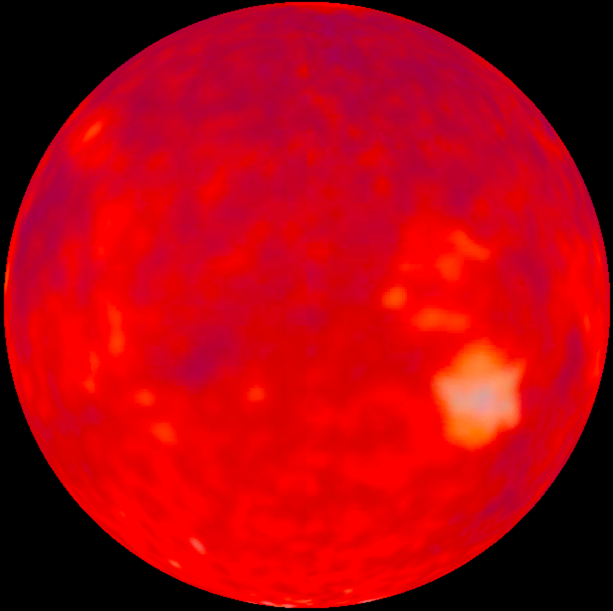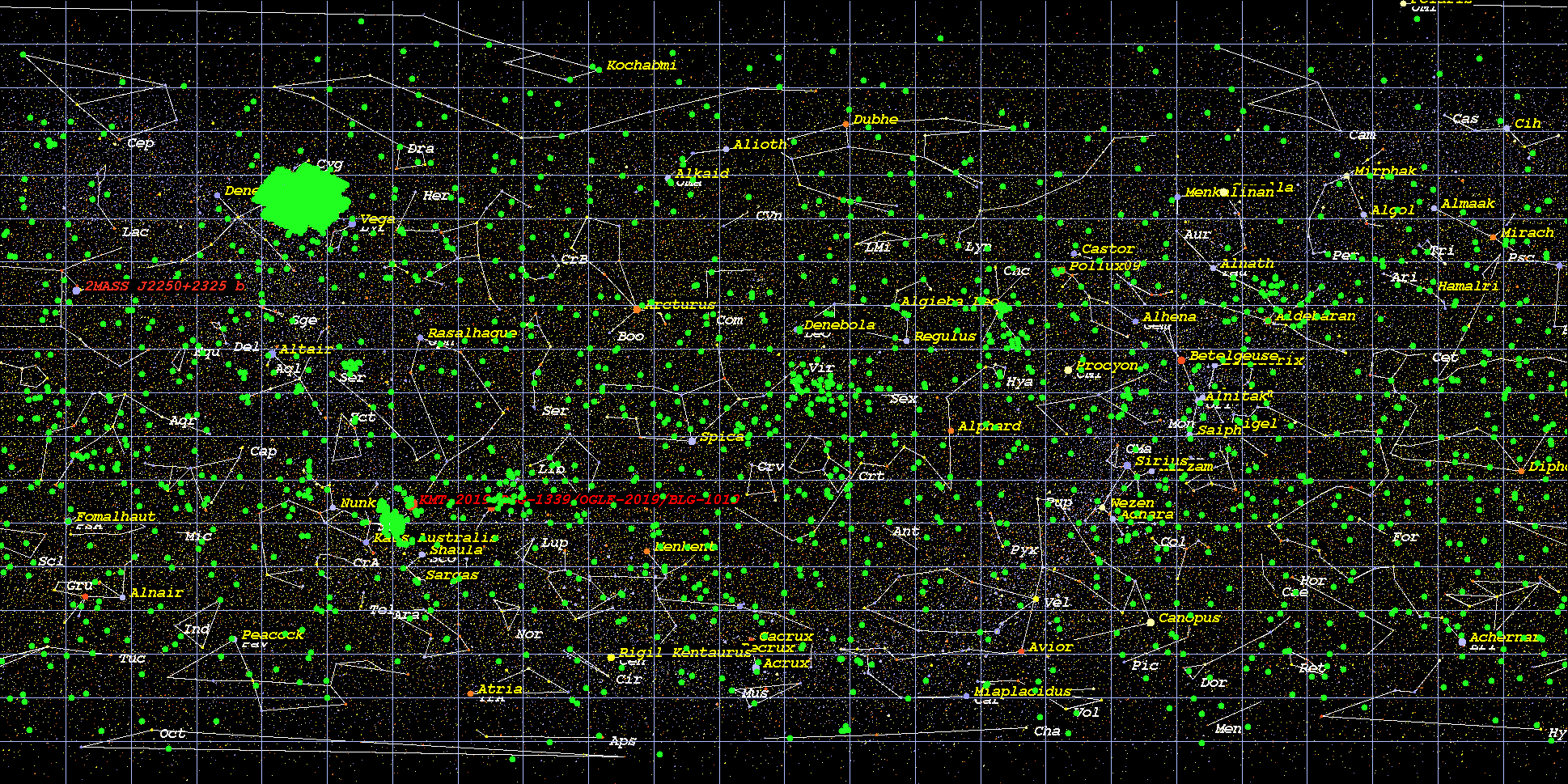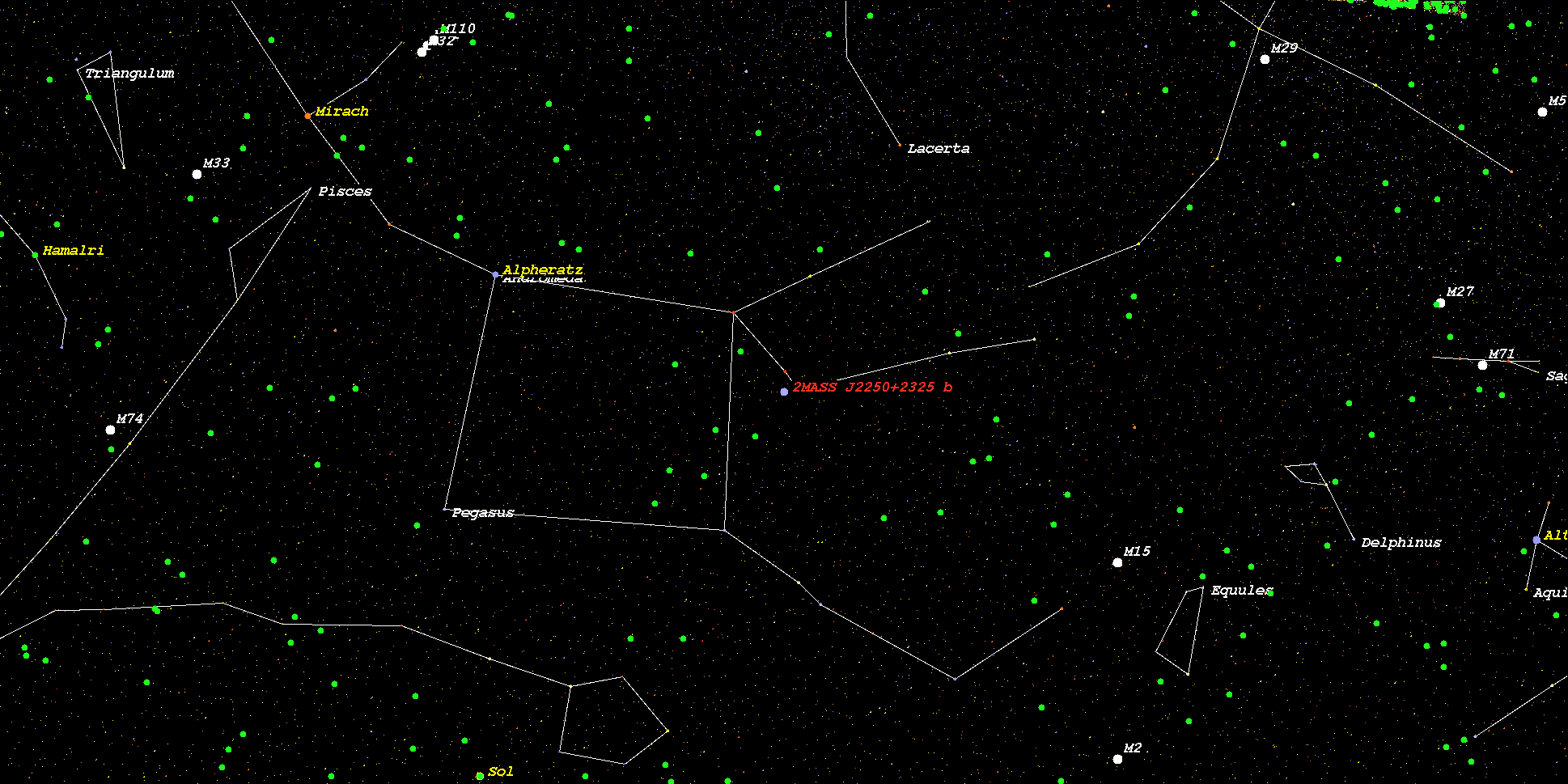2MASS J2250+2325
 Imaginary Picture of Star 2MASS J2250+2325Star Name : 2MASS J2250+2325 Alternate Name : Star Distance (LY/pc): 135.3547 (LY) 41.5000 (pc) Stellar Radius (Rsun): 0.0000 (Rsun, measured) 0.4478 (Rsun, estimated) Stellar Mass (Msun): 0.0000 (Msun/observed) 0.0000 (Msun/estimated) Spectral type : M3 Metalicity : 0.0000 Absolute Magnitude : 18.91 Apparent Magnitude : 22.00 Right Ascension(RA) : 342.56250 Declination(DEC) : 23.42611• This is the 2MASS J2250+2325 . The star 2MASS J2250+2325 islocated about 135.4 light-years (41.5 pc) away from our Solar System.
• The star 2MASS J2250+2325 has apparent magnitude of 22.0, with absolute magnitude of 18.9.
• Number of Extrasolar Planets : 1
• Name of the 1 Planet 2MASS J2250+2325 b radius 0.907830 mass 30.000000 orbital distance 518.000000
(Illustration of the 2MASS J2250+2325's Planetary System)The Star 2MASS J2250+2325 's habitable zone is located at the following distance

Inner Boundary (the orbital distance at Venus's Equivalent Radiation ) : 0.119 AU ( 17778498.1 km)
Earth Boundary (the orbital distance at Earth's Equivalent Radiation) : 0.164 AU ( 24574530.4 km)
Outer Boundary (the orbital distance at Mars's Equivalent Radiation) : 0.250 AU ( 37446146.1 km)
Snow Line (the orbital distance at Snow Line Equivalent Radiation) : 0.368 AU ( 55103644.3 km)
(Habitable zone calculated based on SEAU(Solar Equivalent Astronomical Unit) around the star 2MASS J2250+2325)Kopparapu's Recent Venus for Star 2MASS J2250+2325 distance : 0.134 AU
Kopparapu's Runaway Greenhouse for Earth-sized planet for Star 2MASS J2250+2325 distance : 0.170 AU
Kopparapu's Runaway Greenhouse for Super-Earth-sized planet for Star 2MASS J2250+2325 distance : 0.164 AU
Kopparapu's Runaway Greenhouse for Mars-sized planet for Star 2MASS J2250+2325 distance : 0.179 AU
Kopparapu's Outer Boundary for Maximum Greenhouse for Star 2MASS J2250+2325 distance : 0.331 AU
Kopparapu's Outer Boundary for Early Mars for Star 2MASS J2250+2325 distance : 0.349 AU
(Habitable zone calculated based on Kopparapu et al.(2013) around the star 2MASS J2250+2325)• Original Kopparapu Recent Venus for Star 2MASS J2250+2325 distance : 0.133 AU
• Original Kopparapu Runaway Greenhouse for Star 2MASS J2250+2325 distance : 0.176 AU
• Original Kopparapu Moist Greenhouse for Star 2MASS J2250+2325 distance : 0.176 AU
• Original Kopparapu Outer Boundary for Maximum Greenhouse for Star 2MASS J2250+2325 distance : 0.336 AU
• Original Kopparapu Outer Boundary for Early Mars for Star 2MASS J2250+2325 distance : 0.349 AU

(Habitable zone calculated based on Kopparapu et al.(Original) around the star 2MASS J2250+2325)(Position in Stellar Map of star 2MASS J2250+2325 )(Zoomed position in Stellar Map of star 2MASS J2250+2325 (zoom level 3))